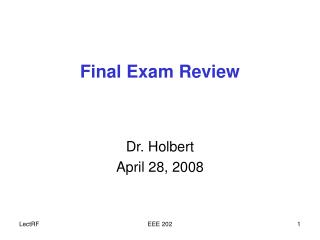DownloadDownload PresentationFinal Exam Review

# Final Exam Review

Download Presentation## Final Exam Review

- - - - - - - - - - - - - - - - - - - - - - - - - - - E N D - - - - - - - - - - - - - - - - - - - - - - - - - - -
##### Presentation Transcript

1. Final Exam Review Dr. Holbert April 28, 2008 EEE 202

2. Don’t Forget the Essentials • Verify voltage polarity and current direction • Obey the passive sign convention • The Fundamentals: Ohm’s Law; KCL; KVL • Series/Parallel Impedance combinations EEE 202

3. Circuit Analysis Techniques • All these circuit analysis techniques have wide applicability: DC, AC, and Transient • Voltage and Current Division • Nodal and Loop/Mesh Analyses • Source Transformation • Superposition • Thevenin’s and Norton’s Theorems EEE 202

4. AC Steady-State Analysis • AC steady-state analysis using phasors allows us to express the relationship between current and voltage using an Ohm’s law-like formula: V = IZ • A phasor is a complex number that represents the magnitude and phase of a sinusoidal voltage or current x(t) = XM cos(ωt+θ) ↔ X = XMθ Time domain Frequency Domain EEE 202

5. Impedance Summary • Z is called impedance(units of ohms, Ω) • Impedance is (often) a complex number, but is not technically a phasor • Impedance depends on frequency, ω EEE 202

6. x is the real part y is the imaginary part z is the magnitude q is the phase angle imaginary axis y z real axis q x Complex Numbers Polar: z q = A = x + jy :Rectangular EEE 202

7. X(j)ejt = X(s)est Y(j)ejt = Y(s)est H(j) = H(s) Transfer Function • Recall that the transfer function, H(s), is • The transfer function in a block diagram form is • The transfer function can be separated into magnitude and phase angle information (s=jω) H(j) = |H(j)| H(j) EEE 202

8. Bode Plots • Place system function in standard form • The terms should appear as: (1 + s) • Magnitude and phase behavior of terms • Constant gain term (K): • with poles/zeros at the origin: find ω0dB • without poles/zeros at origin: use 20 log10(K) dB • Poles and zeros of the form (1 + j) • Sketching the magnitude and phase plots • Reverse: Bode plot to transfer function EEE 202

9. Bode Plot Sketch Summary ωp Gain 0 dB –20 dB ω Phase One Decade 0° –45° –90° ω Pole at ωbreak=1/ EEE 202

10. Bode Plots of Common Filters Gain Gain Low Pass High Pass Frequency Frequency Band Pass Band Reject Gain Gain Frequency Frequency EEE 202

11. Our vocabulary has expanded further with several new terms, including: Resonant frequency Quality factor (Q) Decibels (dB) and decade Active vs. passive filter Phase shift lead/lag RMS current/voltage Bandwidth Break freq., corner freq., cutoff freq., half-power frequency Notch filter Butterworth filter FFT Some Terminology & Quantities EEE 202

12. Course Summary • Bottom line for the semester—can you perform a comprehensive analysis of a given electrical network by determining (as appropriate): • the dc and/or ac output of the circuit • the system response to a step or impulse input • the network transfer function(s) • the system characteristics such as the poles and zeros, and the type of damping exhibited • the frequency response by sketching a Bode plot (magnitude and phase) of the system function • the type of filtering the circuit performs, if any EEE 202